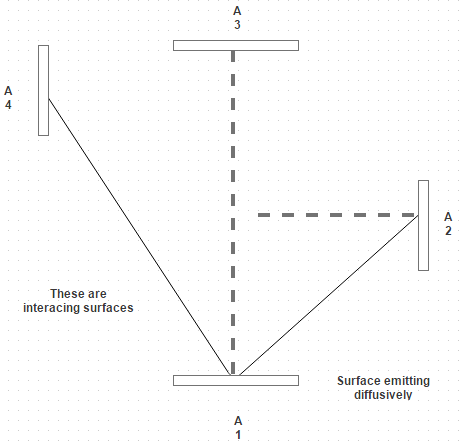## 10 Questions MCQ Test Heat Transfer for Engg. | Test: Intensity Of Radiations

Description
This mock test of Test: Intensity Of Radiations for Chemical Engineering helps you for every Chemical Engineering entrance exam. This contains 10 Multiple Choice Questions for Chemical Engineering Test: Intensity Of Radiations (mcq) to study with solutions a complete question bank. The solved questions answers in this Test: Intensity Of Radiations quiz give you a good mix of easy questions and tough questions. Chemical Engineering students definitely take this Test: Intensity Of Radiations exercise for a better result in the exam. You can find other Test: Intensity Of Radiations extra questions, long questions & short questions for Chemical Engineering on EduRev as well by searching above.
QUESTION: 1

### The solid angle is defined by a region by the rays of a sphere, and is measured as Where, An is projection of incident surface normal to line of propagation.

Solution:

Solid angle is represented by α.

QUESTION: 2

### The plane angle is defined by a region by the rays of a circle, and is measured as\ Where, L is arc of length and r is radius of circle.

Solution:

It is the ratio of arc of length on the circle to the radius of the circle.

QUESTION: 3

### When the incident surface is a sphere, the projection of surface normal to the line of propagation is the silhouette disk of the sphere which is a circle of the diameter of

Solution:

It must be a circle of diameter of a sphere.

QUESTION: 4

If I denotes the normal intensity and I α represents the intensity at angle α, then

Solution:

The intensity of radiation in a direction from the normal is proportional to cosine of the angle.

QUESTION: 5

The intensity of normal radiation I n is how much times the emissive power?

Solution:

= σ T 4/ π and E = σ T 4.

QUESTION: 6

A small surface emits diffusively, and measurements indicate that the total intensity associated with emission in the normal direction I = 6500 W/square m sr. The emitted radiation is intercepted by three surfaces. Mark calculations for intensity associated with emissionSolution:

For a diffusion emitter, the intensity of the emitted radiation is independent of direction.

QUESTION: 7

Consider a deep-space probe constructed as 1 m diameter polished aluminum sphere. Estimate the equilibrium temperature that the probe reaches if the solar energy received is 300 W/m2. For solar radiation, absorptivity of aluminum is 0.3 and the average emissivity appropriate for aluminum at low temperature is 0.04

Solution:

in = α q A P = 70.7 W. Q out = E σ A T 4.

QUESTION: 8

The total emissive power of the emitter with area d A and temperature T is given by

Solution:

E = I π d A.

QUESTION: 9

A black body of 0.2 m2 area has an effective temperature of 800 K. Calculate the intensity of normal radiations

Solution:

I= α T 4/π = 7396.28 W/m2 sr.

QUESTION: 10

The energy radiated out decreases with increases in α and becomes zero at an angle of

Solution:

α = I n cos α. So at 90 degree it becomes zero.